# Power of Powers: Simplifying Exponential Expressions

Lesson Transcript
Instructor
Jennifer Beddoe

Jennifer has an MS in Chemistry and a BS in Biological Sciences.

Expert Contributor
Jenna Strawbridge

Jenna has BA and MS degrees in biological anthropology as well as an MLIS degree in Library & Information Science. She has taught undergraduates for 4 years.

Mathematics is a very logical science. There is a rule for everything and not many exceptions to those rules. Working with exponents is no different. This lesson will describe the rule for raising a power to a power and also will give some examples in how to solve them. Updated: 10/31/2019

## Math Building Blocks

I love Legos. There are so many options! Different colors, sizes, shapes, kits - the possibilities are endless. One day you can build a town, the next day a scene from a movie and the next day you can decide to just pile all the blocks into one free-form structure with no real purpose.

Even though there might not be as much creativity in mathematics as there is in playing with Legos, we end up doing a different kind of building when dealing with numbers and variables. And exponents even have that look of building and expanding. They always remind me of working with blocks.

An error occurred trying to load this video.

Try refreshing the page, or contact customer support.

Coming up next: What Are the Five Main Exponent Properties?

### You're on a roll. Keep up the good work!

Replay
Your next lesson will play in 10 seconds
• 0:07 Math Building Blocks
• 0:42 The Base of an…
• 0:54 The Exponent
• 1:13 Raising a Power to a Power
• 3:08 Lesson Summary
Save Save

Want to watch this again later?

Log in or sign up to add this lesson to a Custom Course.

Timeline
Autoplay
Autoplay
Speed Speed

## The Base of an Exponential Term

The ground floor of any exponential term is called the base.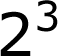In this term, the base is the number 2.

The ground floor of an exponent can be any number or variable.

## The Exponent

The next floor of an exponential term is the exponent - the 3 in this case.

The exponent tells you how many times to multiply a number to itself.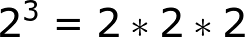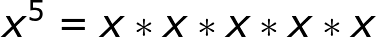## Raising a Power to a Power

As with Legos, there are times when the exponential term has a third floor. This is called raising a power to a power and looks like this: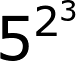These exponential terms can be simplified by writing them out like this, which can then be written as: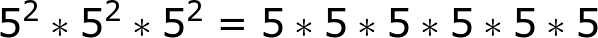which simplifies to 5^6 because there are six 5s being multiplied together.

If we look back to the original problem, we see that by multiplying the two exponents together, we also get 6.

2*3 = 6

So we can assume from this that to simplify a term with a power raised to a power, you just need to multiply the exponents together. But let's try another example.

Simplify: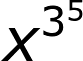To unlock this lesson you must be a Study.com Member.
Create your account

Additional Activities

#### Power Of Powers—Additional Work

At first glance, exponential expressions can appear quite intimidating and difficult to understand. However, there are three simple rules that can make tackling exponential expressions much simpler.

The Product Rule

The product rule is used to multiply exponential expressions with like bases. When an expression has exponential terms with like bases, you can simply add the exponents together. Let's look at an example.

{eq}(x^{3})(x^{4}) {/eq}

{eq}(x^{3+4}) {/eq}

{eq}(x^{3})(x^{4})=(x^{7}) {/eq}

If the coefficients differ between the exponential terms, first multiply the coefficients together. Then, add together the exponents with a common base. Let's look at an example.

{eq}4a^{3}\cdot5a^{2} {/eq}

{eq}20\cdot a^{3+2} {/eq}

{eq}20\cdot a^{5} {/eq}

{eq}4a^{3}\cdot5a^{2}=20a^{5} {/eq}

The Power Rule

The power rule applies to exponents. To simplify a power of a power, you multiply the exponents, keeping the base the same. Let's look at an example.

{eq}(5^{2})^{4} {/eq}

{eq}5^{2\cdot 4} {/eq}

{eq}5^{8}=390,625 {/eq}

The Quotient Rule

The quotient rule for exponents applies when exponential expressions contain division. When you divide two numbers in exponential form with the same base, you can subtract the exponent in the denominator from the exponent in the numerator. Let's look at an example.

{eq}\frac{x^{5}}{x^{2}} {/eq}

{eq}x^{5-2} {/eq}

{eq}x^{3} {/eq}

When dividing terms in an exponential expression that also contain coefficients, divide the coefficients first and then divide the variable powers with the same base by subtracting the exponents. Let's look at an example.

{eq}\frac{15x^4}{5x^2} {/eq}

{eq}(\frac{15}{5})(\frac{x^4}{x^2}) {/eq}

{eq}3(x^{4-2}) {/eq}

{eq}3x^{2} {/eq}

Apply All 3 Rules Together

Now that we've learned how to use the power, product, and quotient rules, let's use all three rules together in an example. Remember, when simplifying an exponential expression, be sure to follow the order of operations. Any easy way to remember the order of operations is the acronym PEMDAS:

Parantheses

Exponents

Multiplication

Division

Addition

Subtraction

Let's work through an example together.

{eq}\frac{x^2(x^5)^3}{8x^8} {/eq}

First, use the power rule to multiply the exponents.

{eq}\frac{x^2(x^{15})}{8x^8} {/eq}

Second, use the product rule to multiply to add together exponents with the same base.

{eq}\frac{x^{17}}{8x^8} {/eq}

Third, use the quotient rule to subtract the denominator's exponent from the numerator's exponent.

{eq}\frac{x^{9}}{8} {/eq}

Here's the full equation reduced to its most simple form.

### Register to view this lesson

Are you a student or a teacher?

### Unlock Your Education

#### See for yourself why 30 million people use Study.com

##### Become a Study.com member and start learning now.
Back
What teachers are saying about Study.com
Create an account to start this course today
Used by over 30 million students worldwide